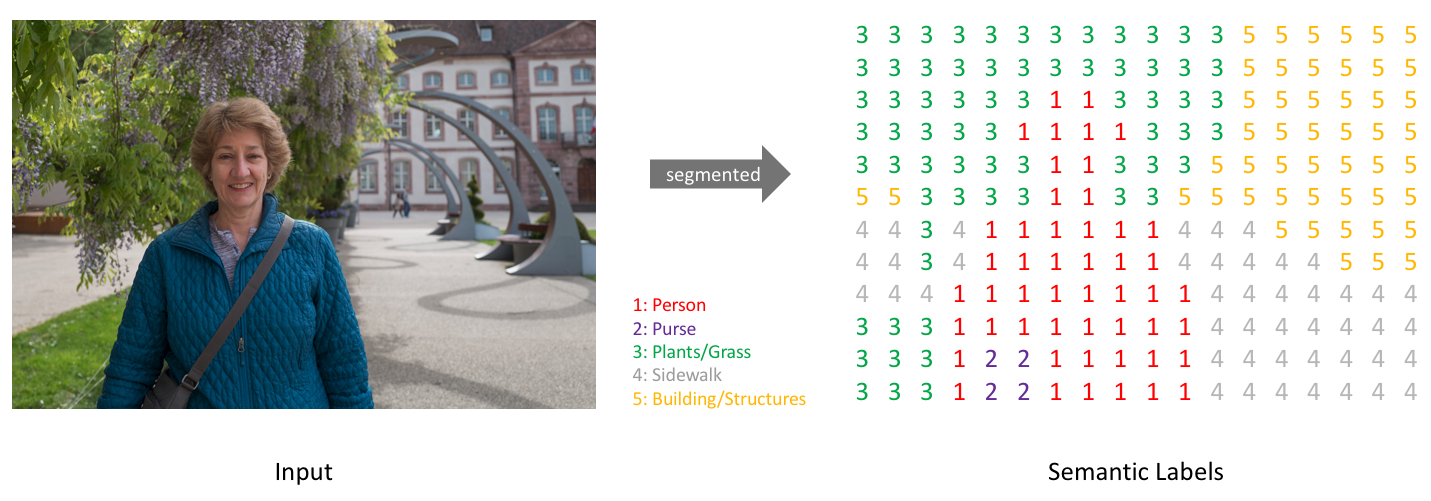# Preprocessing RGB image Masks to Segmentation Masks

Hi, with this kernel, I will transfer your RGB color image masks to pre-process and make it ready for the semantic segmentation model.

The first step in training our segmentation model is to prepare the dataset. We would need the input RGB images and the corresponding segmentation images. If you want to make your own dataset, a tool like labelme or GIMP can be used to manually generate the ground truth segmentation masks. Assign each class a unique ID. In the segmentation images, the pixel value should denote the class ID of the corresponding pixel. You can examine for Deep Learning based Semantic Segmentation exampleIf you have RGB color image masks (like Aerial Semantic Segmentation Drone Dataset RGB_color_masks Folder ) , you can follow the steps below. First of all, our goal is to obtain a pixel-based class image from RGB color image masks as follows.RGB color image maskspixel-based class image masksThe dataset has RGB colored segmentation masks, and there are 24 classes in the dataset. The dataset has a file named class_dict.csv and it contains the name of the classes and their respective RGB values. The contents of the file are as follows:

 name r g b unlabeled 0 0 0 paved-area 128 64 128 dirt 130 76 0 grass 0 102 0 gravel 112 103 87 water 28 42 168 rocks 48 41 30 pool 0 50 89 vegetation 107 142 35 roof 70 70 70 wall 102 102 156 window 254 228 12 door 254 148 12 fence 190 153 153 fence-pole 153 153 153 person 255 22 96 dog 102 51 0 car 9 143 150 bicycle 119 11 32 tree 51 51 0 bald-tree 190 250 190 ar-marker 112 150 146 obstacle 2 135 115 conflicting 255 0 0
``````import os,re
from PIL import Image
import numpy as np
import pandas as pd
import cv2
import matplotlib.pyplot as plt
%matplotlib inline

def atoi(text) :
return int(text) if text.isdigit() else text

def natural_keys(text) :
return [atoi(c) for c in re.split('(\d+)', text)]

read_csv = pd.read_csv('/kaggle/input/semantic-drone-dataset/class_dict_seg.csv',index_col=False,skipinitialspace=True)
read_csv.head()
``````
``````
def segmantation_images(path,new_path, debug_test_num):
filenames = []

for root, dirnames, filenames in os.walk(path):
filenames.sort(key = natural_keys)
rootpath = root

#print(filenames)
count = 0
for item in filenames:

if debug_test_num !=0:
if debug_test_num <= count:
break

count = count + 1

if os.path.isfile(path+item):
f, e = os.path.splitext(item)
image_rgb = Image.open(path+item)
image_rgb = np.asarray(image_rgb)
new_image = np.zeros((image_rgb.shape,image_rgb.shape,3)).astype('int')

for index, row  in read_csv.iterrows():
new_image[(image_rgb[:,:,0]==row.r)&
(image_rgb[:,:,1]==row.g)&
(image_rgb[:,:,2]==row.b)]=np.array([index+1,index+1,index+1]).reshape(1,3)

new_image = new_image[:,:,0]
output_filename = new_path+f+'.png'
cv2.imwrite(output_filename,new_image)
print('writing file: ',output_filename)

else:
print('no file')

print("number of files written: ",count)
``````
``````debug_test_num = 5 # 5 samples are enough just to see it working. If you set 0, you can do all the datasets.
segmantation_images("/kaggle/input/semantic-drone-dataset/RGB_color_image_masks/RGB_color_image_masks/", "", debug_test_num=debug_test_num)
``````
``````from PIL import Image
input_image = "/kaggle/input/semantic-drone-dataset/RGB_color_image_masks/RGB_color_image_masks/"+"002.png"
output_image = "002.png"

fig, axs = plt.subplots(1, 2, figsize=(16, 8), constrained_layout=True)

img_orig = Image.open(input_image)
axs.imshow(img_orig)
axs.grid(False)

output_image = Image.open(output_image)
out_array = np.asarray(output_image)
axs.imshow(out_array)
axs.grid(False)
``````